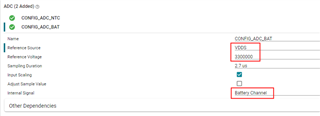If you have a related question, please click the "Ask a related question" button in the top right corner. The newly created question will be automatically linked to this question.

Part Number: LP-CC2652RB
Other Parts Discussed in Thread: CC2652RB

Hi Ti:1. reference source 选 Fixed / VDDS 哪个精度更高呢？ Fixed 的参考电压是多少呢？ 采样电压范围是多少呢？

2. Input Scaling 这个参数是什么意思呢？ 我看到其他 ADC 设置中有 Gain 这个参数，是类似的意思吗？

• 1，2.如果VDDS是恒压源，那二者精度是差不多的

是的，具体参考下面的说明

• In practice, using the internal fixed voltage reference sets the upper range of the ADC to a fixed value. That value is 4.3V with input scaling enabled and ~1.4785V with input scaling disabled. In this mode, the output is a function of the input voltage multiplied by the resolution in alternatives (not bits) divided by the upper voltage range of the ADC. Output = Input (V) * 2^12 / (ADC range (V))
• Using VDDS as a reference scales the upper range of the ADC with the battery voltage. As the battery depletes and its voltage drops, so does the range of the ADC. This is helpful when measuring signals that are generated relative to the battery voltage. In this mode, the output is a function of the input voltage multiplied by the resolution in alternatives (not bits) divided by VDDS multiplied by a scaling factor derived from the input scaling. Output = Input (V) * 2^12 / (VDDS (V) * Scaling factor), where the scaling factor is ~1.4785/4.3 for input scaling disabled and 1 for input scaling enabled.

3.一般不用这个,CC2652RB采样值基本上是很准确的

• Hi,

根据你的回答，已经大致解决了我的疑问，不过对于如下配置还有一点疑问：

配置参考下图，假设用 3.3V 电池进行供电，在刚开始时，电池电压很接近下图的设置值，但是工作一段时间后，电池电压下降了，那么测得的结果是不是会更不准确了（会比实际值偏高吗）？可以把上次回答所引用的英文文档链接分享一下吗？

谢谢你！